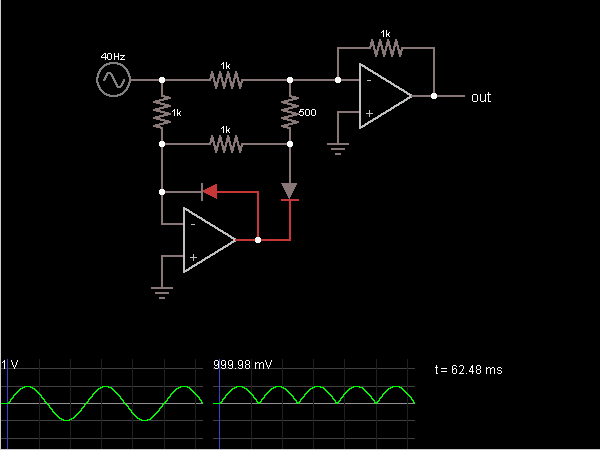# Circuit Simulator - Full-Wave Rectifier (with op-amps)

Full-Wave Rectifier (with op-amps)Circuit Description:
This is a full-wave rectifier, superior to the simple diode version because there is no 700 mV diode drop.

The lower left op-amp attempts to keep its – input at the same voltage as the + input (which is at ground). When the input signal is negative, the op-amp output goes positive to keep the – input at ground. The 500 ohm resistor has no current flowing through it, because both ends are at ground, so it can be ignored. The second op-amp and two 1k resistors act as a unity-gain inverting amplifier to make the output equal to the input, only positive.

When the input signal is positive, both op-amps attempt to keep their – input at ground. In order to do this, all four resistors on the left must have a voltage drop equal to the input signal. The 500 ohm resistor must have twice as much current flowing through it as the 1k resistor to its upper left (because it has the same voltage drop but half the resistance), so the upper right resistor must make up the difference. This requires the ciruit's output to be at the same level as the input signal, with the same polarity.

So the output voltage is always positive but has the same magnitude as the input voltage.

Discussion:
2 comments Page 1 of 1.

Helloworld said:   7 years ago
How can I smooth out this circuit to get a straight dc output?
(3)

Tech. said:   8 years ago
How would I go about filtering an output waveform as such? Would a smoothing capacitor be effective in such circumstances?
(2)